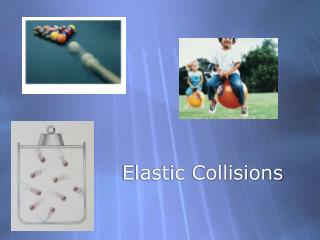# Elastic Collisions - PowerPoint PPT PresentationDownload PresentationElastic Collisions

Elastic Collisions
Download Presentation## Elastic Collisions

- - - - - - - - - - - - - - - - - - - - - - - - - - - E N D - - - - - - - - - - - - - - - - - - - - - - - - - - -
##### Presentation Transcript

1. Elastic Collisions

2. 1. A cart collides and sticks to a stationary cart of equal mass and they both travel at speed v. If the cart were to instead bounce off the stationary cart, the stationary cart would move at a speed greater than v less than v equal to v equal to zero don’t know

3. 2. Ball 1 collides with speed v with the 4 balls. All balls are the same mass. Which of the following outcomes would satisfy conservation of momentum? Ball 1 stops, one ball comes out at v Ball 1 stops, two balls come out at v/2 Ball 1 stops, three balls come out at v/3 All of the above None of the above 1

4. 3. Ball 1 collides with speed v with the 4 balls. All balls are the same mass. Which of the following outcomes would satisfy conservation of energy? Ball 1 stops, one ball comes out at v Ball 1 stops, two balls come out at v/2 Ball 1 stops, three balls come out at v/3 All of the above None of the above 1

5. 4. Bowling ball 1 collides with stationary bowling ball 2 of equal mass. Which of the following outcomes satisfies the conservation of momentum and conservation kinetic energy? Ball 1 stops, ball 2 has speed v Ball 1 slows to v/2, ball 2 has speed v/2 Ball 1 slows to v/3, ball 2 has speed v/3 All of the above None of the above 1 2

6. 5. 2 carts of equal mass and equal speed collide with each other and then stick together. Which of the following is true? The momentum is the same before and after. The kinetic energy is the same before and after. The kinetic energy is zero before the collision. The momentum is 2mv before the collision. All of the above

7. 6. 2 carts of equal mass and equal speed collide with each other and then bounce in an elastic collision. Which of the following is true? The momentum is the same before and after. The kinetic energy is the same before and after. The momentum is zero after the collision. The momentum is zero before the collision. All of the above

8. 7. 2 carts of equal mass and equal speed v collide with each other and then bounce in an elastic collision. What is the final velocity of the cart on the left? v/2 v -v/2 -v -2v

9. 8. A cart of mass 2m and speed v collides with a stationary cart of mass m in an elastic collision. What is the final velocity of the cart on the right? v/3 v/2 2v/3 4v/3 3v/2

10. 9. Two people on skateboards throw a ball back and forth. After a couple of throws, ignoring friction, they are: A. standing where they were initially. B. standing farther away from each other. C. standing closer together. D. moving away from each other. E. moving toward each other.

11. 10. Suppose you are on a cart, initially at rest on a track with very little friction. You throw balls at a partition that is rigidly mounted on the cart. If the balls bounce straight back as shown in the figure, is the cart put in motion? A. Yes, it moves to the right. B. Yes, it moves to the left. C. No, it remains in place.

12. 11. True or False: A stationary object that is struck by a moving object can have a larger final momentum than the initial momentum of the incoming object.

13. True or False: A stationary object that is struck by a moving object can have a larger final momentum than the initial momentum of the incoming object? True, consider an incoming object with an initial momentum mv hitting an object of mass m. It bounces backwards with almost the same speed. It now has a momentum of -mv. Since the momentum can’t change, the stationary object must now have a momentum of 2mv. pi = pf mv = -mv + 2mv

14. 12. A cart with a spring plunger runs into a fixed barrier. Rank the following situations from least to greatest according to the change in momentum of the car. + A and D tie, C, D D, B and C tie, A D, A and C tie, B D and C tie, A, B B and D tie, C, A